# Circumscribed circle to square

Find the length of a circle circumscribing a square of side 10 cm. Compare it to the perimeter of this square.

d =  0 cm

### Step-by-step explanation: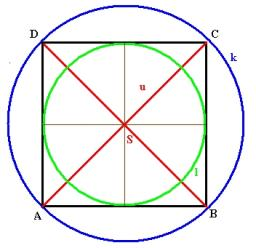Did you find an error or inaccuracy? Feel free to write us. Thank you!Tips to related online calculators
Pythagorean theorem is the base for the right triangle calculator.

#### You need to know the following knowledge to solve this word math problem:

We encourage you to watch this tutorial video on this math problem:

## Related math problems and questions:

• Find the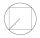Find the length of the side of the square ABCD, which is described by a circle k with a radius of 10 cm.
• Rectangle - desc circle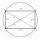Length of the sides of the rectangle are at a ratio 1: 3 . Radius of the circle circumscribed to rectangle is 10 cm. Calculate the rectangle's perimeter.
• Rhombus and inscribed circleIt is given a rhombus with side a = 6 cm and the radius of the inscribed circle r = 2 cm. Calculate the length of its two diagonals.
• Circumscribing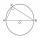Determine the radius of the circumscribed circle to the right triangle with legs 6 cm and 3 cm.
• RectangleThe rectangle is 21 cm long and 38 cm wide. Determine the radius of the circle circumscribing rectangle.
• Rectangle and circle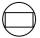The rectangle ABCD has side lengths a = 40 mm and b = 30 mm and is circumscribed by a circle k. Calculate approximately how many cm is circle long.
• RhombusFind the length of the other diagonal and area of the rhombus. The perimeter of a rhombus is 40 cm and one of the diagonals is of length 10 cm.
• Rectangle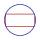The rectangle is 18 cm long and 10 cm wide. Determine the diameter of the circle circumscribed to the rectangle.
• Side and diagonalFind the circumference and the area of the rectangle if given: side a = 8 cm diagonal u = 10 cm.
• Square diagonalCalculate the length of the square diagonal if the perimeter is 172 cm.
• Square circles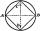Calculate the length of the described and inscribed circle to the square ABCD with a side of 5cm.
• Circle chordCalculate the length of the chord of the circle with radius r = 10 cm, length of which is equal to the distance from the center of the circle.
• DiagonalsA diagonal of a rhombus is 20 cm long. If it's one side is 26 cm, find the length of the other diagonal.
• Diagonal of squareCalculate the side of a square when its diagonal is 10 cm.
• Diagonal - simple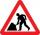Calculate the length of the diagonal of a rectangle with dimensions 5 cm and 12 cm.
• CirclesThe area of a circle inscribed in a square is 14. What is the area of a circle circumscribed around a square?
• DiagonalCalculate the diagonal length of the rectangle ABCD with sides a = 8 cm, b = 7 cm.